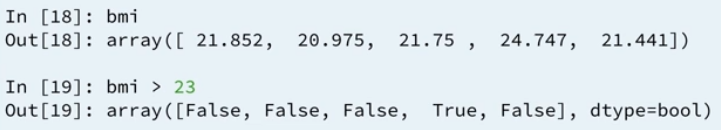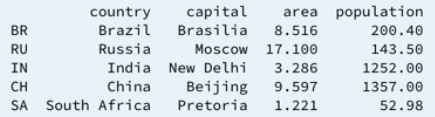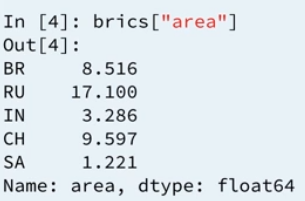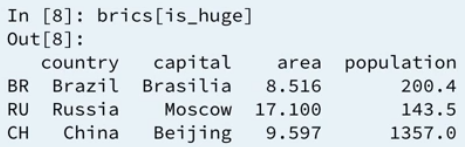# Intermediate Python for Data Science: Logic, Control Flow and FilteringBoolean logic is the foundation of decision-making in Python programs. Learn about different comparison operators, how to combine them with Boolean operators, and how to use the Boolean outcomes in control structures. Also learn to filter data in pandas DataFrames using logic.

In the earlier days when I started to learn Python, there is a topic on Boolean and Comparison Operators, where I studied Boolean (True and False), logical operators (‘and’, ‘or’, ‘not’) and comparison operators (‘==’ ‘!=’, ‘<‘ and ‘>’).

Comparison operators can tell how two Python values relate and result in a Boolean. It allows to compare two numbers, strings or any same type of variables. It throws exception or error message when it is comparing a variable from a different data type. Python cannot tell how the two objects of different type relate.

## Comparison a Numpy array with an integerBased on the example above taken from a tutorial in DataCamp online learning course that I am taking currently, the variable bmi is a Numpy array, then it compares if the bmi is greater than 23. It works perfectly and returns the Boolean values. Behind the scenes, Numpy builds a Numpy array of the same size, perform an element-wise comparison, filtered with the number 23.

## Boolean operators with Numpy

To use these operators with Numpy, you will need np.logical_and(), np.logical_or() and np.logical_not(). Here’s an example on the my_house and your_house arrays from before to give you an idea:

```np.logical_and(your_house > 13,
your_house < 15)
```

Refer to below for the sample code:

```# Create arrays
import numpy as np
my_house = np.array([18.0, 20.0, 10.75, 9.50])
your_house = np.array([14.0, 24.0, 14.25, 9.0])

# my_house greater than 18.5 or smaller than 10
print(np.logical_or(my_house > 18.5, my_house < 10))

# Both my_house and your_house smaller than 11
print(np.logical_and(my_house < 11, your_house < 11))
```

The first print statement is checking on the ‘or’ condition means, any one of the two condition is true, it returns true. The second print statement is checking on the ‘and’ condition means, both of the comparison has to be True then it returns a True. The output of the execution returns in Boolean array as below:

```[False  True False  True]
[False False False  True]
```

## Combining Boolean operators and Comparison operators with conditional statement, if, else and elif.

It follows the if statement syntax. The most simplest code which can be used to explain the above,

```z = 4
if z % 2 == 0:
print('z is even')
```

Same goes to the if else statement with comparison operator, see code below:

```z = 5
if z % 2 == 0:
print('z is even')
else:
print('z is odd')
```

Or if you are working with if, elif and else statement, it works too. See the code below:

```z = 6
if z % 2 == 0:
print('z is divisible by 2')
elif z % 3 == 0:
print('z is divisible by 3')
else:
print('z is neither divisible by 2 nor 3')
```

In the example above, both first and second condition are matched, however, in this control structure, once Python hits into a condition that returns a True value, it executes the corresponding code and exits the control structure after that. It will not execute the next condition, corresponding to the elif statement.

## Filtering Pandas DataFrame

For an example taken from DataCamp’s tutorial, using the DataFrame below, select countries with area over 8 millions km. There are 3 steps to achieve this.Step 1: select the area column from the DataFrame. Ideally, it gets a Pandas Series, not a Pandas DataFrame. Assume that the DataFrame is called bric, then it calls the column area using,

```brics["area"]

#alternatively it can use the below too:
# brics.loc[:, "area"]
# brics.iloc[:, 2]
```Step 2: When the code adds in the comparison operator to see which rows have an area greater than 8, it returns a Series containing Boolean values. The final step is using this Boolean Series to subset the Pandas DataFrame.

Step 3: Store this Boolean Series as ‘is_huge’ as below:

```is_huge = brics["area"] > 8
```

Then, creates a subset of DataFrame using the following code and the result returns as per the screenshot:

```brics[is_huge]
```It shows those countries with ares greater than 8 million km. The steps can be shorten into 1 line of code:

```brics[brics["area"] > 8]
```

Also, it is able to work with Boolean operators (np.logical_and(), np.logical_or() and np.logical_not()). For example, if it looks for areas between 8 and 10 km, then the single line code can be:

```brics[np.logical_and(brics["area"] > 8, brics["area"] < 10)]
```

The result returns from the above code is Brazil and China.